Courses

# Radioactivity Introduction (Part-1) Chemistry Notes | EduRev

## Chemistry : Radioactivity Introduction (Part-1) Chemistry Notes | EduRev

The document Radioactivity Introduction (Part-1) Chemistry Notes | EduRev is a part of the Chemistry Course Physical Chemistry.
All you need of Chemistry at this link: Chemistry

1. Radioactivity: Radioactive decay (also known as nuclear decay or radioactivity) is the process by which an unstable atomic nucleus loses energy (in terms of mass in its rest frame) by emitting radiation, such as an alpha particle, beta particle with neutrino or only a neutrino in the case of electron capture, gamma ray, or electron in the case of internal conversion. A material containing such unstable nuclei is considered radioactive. Certain highly excited short-lived nuclear states can decay through neutron emission, or more rarely, proton emission.

Radioactive decay is a stochastic (i.e. random) process at the level of a singular quantum of single atoms, in that, according to quantum theory, it is impossible to predict when a particular atom will decay, regardless of how long the atom has existed. However, for a collection of atoms, the collection's expected decay rate is characterized in terms of their measured decay constants or half-lives. This is the basis of radiometric dating. The half-lives of radioactive atoms have no known upper limit, spanning a time range of over 55 orders of magnitude, from nearly instantaneous to far longer than the age of the universe.

All the heavy elements possess radioactive properties. However, the radioactive property of the different radioactive elements differs widely, e.g. radium atoms have about three million times the activity of uranium atoms. Uranium in the form of potassium uranyl sulfate, KUO2(SO4)2 was the first compound found to be radioactive. Radioactive changes are spontaneous. These are not controlled by temperature, the pressure of nature of chemical combination.

2. Radioactive disintegration The atomic nuclei of radioactive elements can disintegrate any moment. During disintegration, atoms of new elements having different physical and chemical properties are formed, called daughter elements.

Disintegration occurs by the following processes:

(i) α-particle emission: When an a-particle is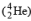emitted from the nucleus of the parent element, the new element formed called daughter element, possess atomic mass less by 4 unit & atomic number less by 2 units.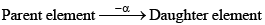Atomic mass      A        A - 4

Atomic number Z        Z - 2

(ii) β-particle emission: When β-particle is emitted from parent element thus formed daughter elements possess possesses same atomic mass but atomic no. is increased by 1 unit.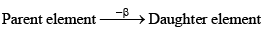Atomic mass       A      A

Atomic number  Z      Z + 1

· Elements having the same mass number called isobars. (A-same, Z-different)

∴ daughter elements formed by the β-particle emission is an isobar of the parent element.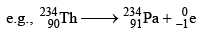If in a radioactive transformation α & β both are emitted then atomic mass & atomic number changes accordingly & produces an isotope of the parent element.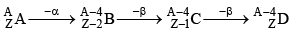(A & D) are isotopes)

3. Some important terms:

· Element having same no. of protons called Isotopes. e.g.,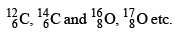(A different, Z-different)

· Elements having the same no. of neutrons are called Isotones e.g.,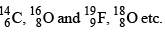(A different, Z-different)

· Elements having the same value of (A-2Z) are called Isodiaphers.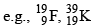(A different, Z-different, A-2Z-same)

· Elements having the same number of electrons are called Isoelectronic. e.g.,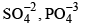· Compounds having the same number of atoms as well as the same number of electrons are called Isosteres.

(i) Atoms of all radioactive elements undergo spontaneous disintegration and form new radioactive elements. The disintegration is accompanied by the emission of α, β or γ-rays.
(ii) The disintegration is at random, i.e., every atom has an equal chance for disintegration any time.
(iii) The number of atoms that disintegrate per second is directly proportional to the number of remaining unchanged radioactive atoms present at any time. The disintegration is independent of all physical and chemical conditions like temperature, pressure chemical combination etc. The two laws of radioactive disintegration can be summed up as below:

1. Group displacement Law: The result of α-and β-particle changes can be summed up in the form of group-displacement law. "In an α-particle change the resulting element has an atomic weight less by four units and atomic number less by two units and it falls in a group of the periodic table two columns to the left of the original element, and in α β-particle change the resulting element has same atomic weight but its atomic number is increased by one than its parent and hence it lies one column to right."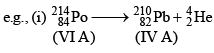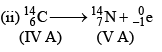2. Law of radioactivity decay: According to the law of radioactive decay, the quantity of a radioelement which disappears in unit time (rate of disintegration) is directly proportional to the amount present.

5. Determination of the number of α-and β-particles emitted in a nuclear reaction: Consider the following general reaction.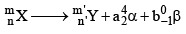Then, m = m’ + 4a + 0b (ii) n = n’ + 2a – b Solve for a and b

Where a is the number of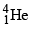emitted and b is the of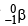emitted.

6. Points to Remember

1. The rate of decay (activity, A) is the number of atoms undergoing decay to unit time; it is represented by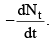2. The rate of decay of a nuclide is directly proportional to the number of atoms of that nuclide present at that moment, hence.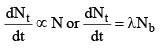(the negative sign shows that the number of radioactive atoms, Nt decreases as time t increases)

3. The rate of decay of nuclide is independent of temperature, so its energy of activation is zero.

4. Since the rate decay is directly proportional to the amount of the radioactive nuclide present and as the number of unrecompensed atoms decreases with increase in time, the rate of decay also decreases with the increase in time.

Various forms of equation for radioactive decay are

Nt = N0e–λt

log N0 - log Nt = 2.303 λt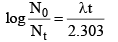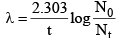..........(1)

Note that the equation (I) is similar to that of first order reaction, hence we can say that radioactive disintegration is examples of first order reactions.

Where N0 = Initial number of atoms of the gives nuclide, i.e. at time 0

Nt = No. of atoms of that nuclide present, i.e. at time 0

λ = Decay constant

However, unlike first-order rate constant (K), the decay constant (λ) is independent of temperature.

7. The decay constant: The ratio between the number of atoms disintegrating in unit time to the total number of atoms present at that time is called the decay constant of that nuclide.

Characteristics of decay constant (λ)

1. It is characteristic of a nuclide (not of an element)

2. Its units are time-1

3. Its value is always less than one

8. Half-life Period (T1/2 or t1/2): Rutherford in 1904 introduced a constant known as half-life period of the radio-element for evaluating its radioactivity or for comparing its radioactivity with the activities of other elements. The half-life period of radio-element is defined as the time required by a given amount of the element of decay to one-half of its initial value.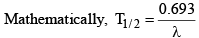9. Average Life Period (T): Since total decay of any element is infinity. It is meaningless to use the term total decay period (total life period) for radio elements. Thus the term average life is used which is used which determined by the following relation.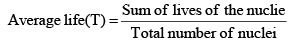The relation between average life and half-life. Average life (T) of an element is the inverse of its

Decay constant i.e., T = 1/λ

Substituting the value of λ in the above equation,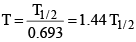Thus, Average life (T) = 1.44 × Half-life (T1/2)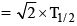Thus the average life period of a radioisotope is approximately under-root two times of its half-life period.

10. Specific Activity: It is the measure of radioactivity of a radioactive substance. It is defined as ‘te number of radioactive nuclei which decay per second per gram of radioactive isotope’. Mathematically, if’ is the mass of radioactive isotope. Then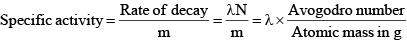Where N is the number of radioactive nuclei, which undergoes disintegration.

(a) The phenomenon of spontaneous disintegration of nuclei of unstable atoms is defined as radioactivity.
(b) Generally, it is exhibited by atoms with A > 192 and Z > 82
(c) It was discovered by Henry Becquerel
(d) Lead isotope is the stable and product of any natural radioactive series
(e) Radioactivity is a nuclear process and not an atomic process.
(f) Radioactivity is not associated with the electron configuration of the atom.

12. Rutherford-Soddy Theory:

(a) If N is the number of radioactive nuclei present in a sample at a given instant of time, then the rate of decay at that instant is proportional to N i.e.,

dN/dt = -λN

(b) If N0 is the number of radioactive nuclei at time t = 0, then the number of radioactive nuclei at a later time t is given by, N = N0e–λt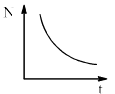(c) The nuclei of unstable atoms decay spontaneously emitting α, β particles, and γ-rays
(d) Radioactivity remains unaffected due to the physical and chemical changes of the material.
(e) Radioactivity obeys the law of probability i.e. it is uncertain that when a particular atom will decay.

13. Soddy-Fajaan’s Laws:

(a) During an α-decay, the mass number decreases by 4 units and atomic number 2 units.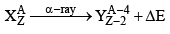Daughter nucleus will occupy two positions before that of parent nucleus, in the periodic table.

(b) During β-decay, the mass number of the atom will not change and the atomic number increases by 1 unit.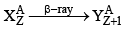Daughter nucleus will occupy one position on the right of that of parent nucleus in the periodic table

(c) During γ-decay, the mass number and atomic number of the nucleus remain unchanged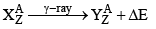(d) Emission of a-part ice means loss of two protons and two neutrons
(e) Emission of ²-particle means loss of an electron
(f) Emission of a ³-ray means no change in charge and mass, but only energy changes.

14. Activity (A):

(a) The number of atoms of any material decaying per second is defined as the activity of that material.
(b) Its value depends on the quantity and nature of that material.
(c) Units of activity Fundamental unit- disintegrations per second i.e., Bq

1 Bq = 1disintegration/s
(d) Practical unit s: curie and Rutherford.

1 curie = 3.7 × 1010 disintegration/second:

1 Rutherford = 106 disintegrations/second.

(e) Formulae of activity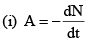(ii) A = λN
(iii) A0 = λN0
(iv) A = A0e-λt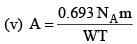Where A0 = maximum initial activity; A = activity after time t, λ = decay constant.

NA = Avogadro number, m = mass of material

W = Atomic weight of the material, T = half-life of the material

15. Decay Constant (λ):

(a) The decay constant is equal to the reciprocal of that time in which the activity of the material reduces to 1/e or 37% of its initial activity.

(b)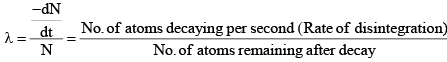i.e. The rate of disintegration per atom is defined as decay constant

(c) Decay constant does not depend on temperature, pressure, and volume. It depends on the nature of the material.
(d) It's unit per second.

(e) Decay constant represents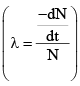the probability of decay per second.

(f)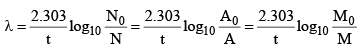(g) Its value is equal to the negative of the slope of N-t curve.

(h) The decay constant of the stable element is zero.

Offer running on EduRev: Apply code STAYHOME200 to get INR 200 off on our premium plan EduRev Infinity!

## Physical Chemistry

66 videos|94 docs|32 tests

,

,

,

,

,

,

,

,

,

,

,

,

,

,

,

,

,

,

,

,

,

;